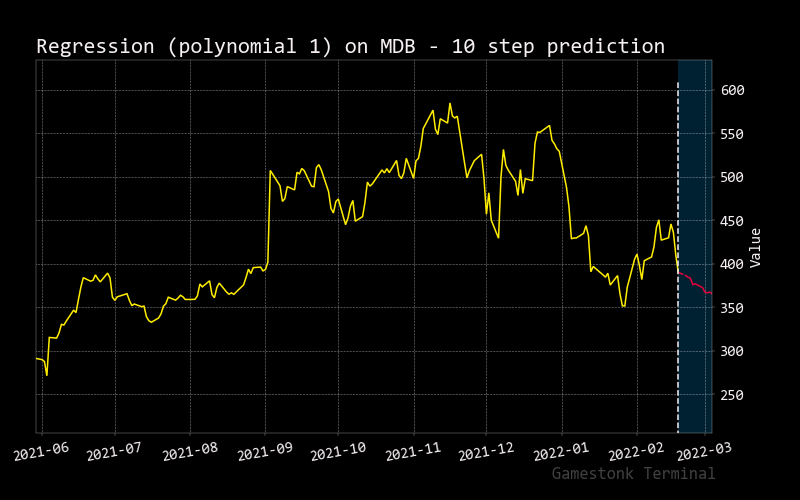# Regression

``````usage: regression [-i N_INPUTS] [-d N_DAYS] [-j N_JUMPS] [-e S_END_DATE] [-p N_POLYNOMIAL] [-h]
[--export {png,jpg,pdf,svg}]
``````

Regression attempts to model the relationship between two variables by fitting a linear/quadratic/cubic/other equation to observed data. One variable is considered to be an explanatory variable, and the other is considered to be a dependent variable.

``````optional arguments:
-i N_INPUTS, --input N_INPUTS
number of days to use for prediction.
-d N_DAYS, --days N_DAYS
prediction days.
-j N_JUMPS, --jumps N_JUMPS
number of jumps in training data.
-e S_END_DATE, --end S_END_DATE
The end date (format YYYY-MM-DD) to select - Backtesting
-p N_POLYNOMIAL, --polynomial N_POLYNOMIAL
polynomial associated with regression.
-h, --help            show this help message
--export {png,jpg,pdf,svg}
Export figure into png, jpg, pdf, svg
``````